### Araf by Joseph Howard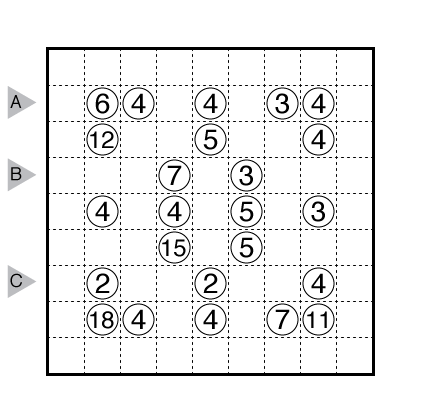PDF

Theme: Clue Symmetry & Logic

Author/Opus: This is the 1st puzzle from guest contributor Joseph Howard.

Rules: Standard Araf rules.

Answer String: Enter the number of cells in each connected group (between bold lines) in the marked rows.

Time Standards (highlight to view): Grandmaster = 3:45, Master = 6:15, Expert = 12:30

Note: Follow this link for more Araf puzzles on this website. If you are new to this puzzle type, here are our easiest Araf Puzzles to get started on.

### Araf by Hans van Stippent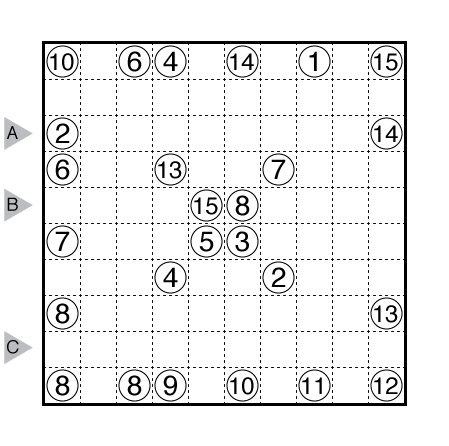PDF

Theme: X Frame

Author/Opus: This is the 7th puzzle from guest contributor Hans van Stippent.

Rules: Standard Araf rules.

Answer String: Enter the number of cells in each connected group (between bold lines) in the marked rows.

Time Standards (highlight to view): Grandmaster = 2:30, Master = 3:30, Expert = 7:00

Note: Follow this link for more Araf puzzles on this website. If you are new to this puzzle type, here are our easiest Araf Puzzles to get started on.

### Araf by Serkan Yürekli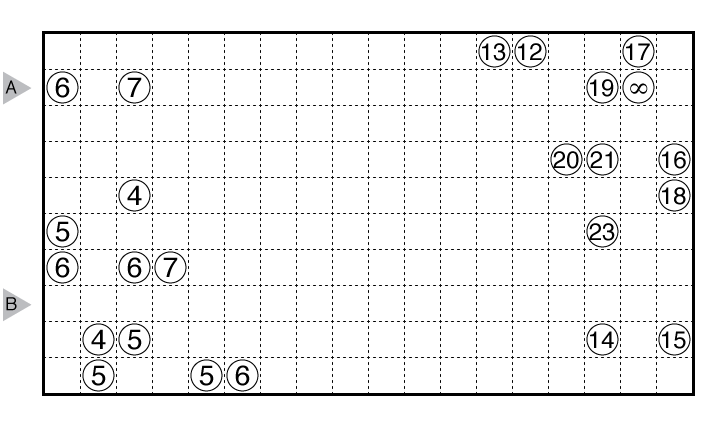(view image directly for larger form)

PDF

Theme: Infinity!

Author/Opus: This is the 51st puzzle from our contributing puzzlemaster Serkan Yürekli.

Rules: Divide the grid into some regions formed of edge-adjacent squares. Each cell is part of one region, and each region should contain exactly two given numbers. Each region must have an area that is strictly between those numbers (This means, for two number clues A and B with A < B, the area C fulfills A < C < B).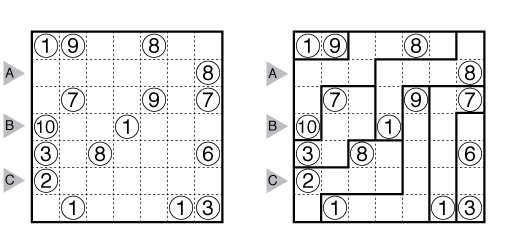Answer String: Enter the number of cells in each connected group (between bold lines) in the marked rows. If a group is a multi-digit number, enter all digits of that number. For the example above, the entry is “34,121111,4111”.

Time Standards (highlight to view): Grandmaster = 8:00, Master = 15:00, Expert = 30:00

Note: Araf is a relatively new puzzle for us on GMPuzzles. Follow this link for other Araf.

### Araf by Bram de Laat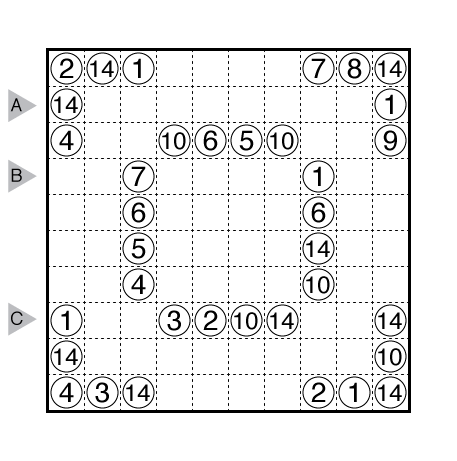PDF

Theme: Clue Symmetry and Logic

Author/Opus: This is the 4th puzzle from guest contributor Bram de Laat.

Rules: Divide the grid into some regions formed of edge-adjacent squares. Each cell is part of one region, and each region should contain exactly two given numbers. Each region must have an area that is strictly between those numbers (This means, for two number clues A and B with A < B, the area C fulfills A < C < B).Answer String: Enter the number of cells in each connected group (between bold lines) in the marked rows. For the example above, this is “34,121111,4111”.

Time Standards (highlight to view): Grandmaster = 4:40, Master = 6:00, Expert = 12:00

Note: Araf is a relatively new puzzle for us on GMPuzzles. Follow this link for other Araf.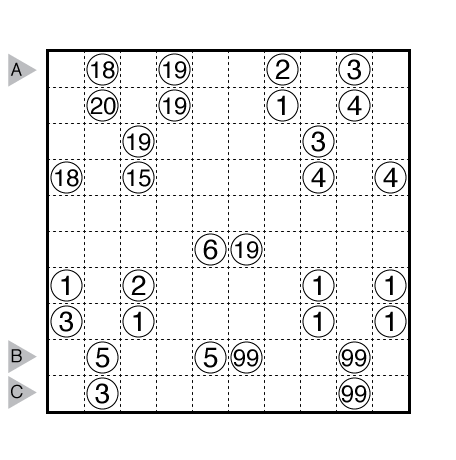PDF

Theme: Four Y Intersections

Author/Opus: This is the 24th puzzle from our contributing puzzlemaster Prasanna Seshadri.

Rules: Divide the grid into some regions formed of edge-adjacent squares. Each cell is part of one region, and each region should contain exactly two given numbers. Each region must have an area that is strictly between those numbers (This means, for two number clues A and B with A < B, the area C fulfills A < C < B).Answer String: Enter the number of cells in each connected group (between bold lines) in the marked rows. For the example above, this is “34,121111,4111”.

Time Standards (highlight to view): Grandmaster = 3:15, Master = 5:00, Expert = 10:00

Note: Araf is a relatively new puzzle for us on GMPuzzles. Follow this link for other Araf.

### Araf by Serkan Yürekli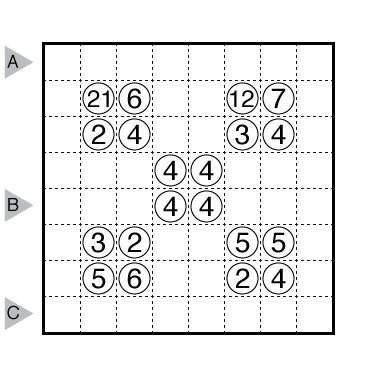PDF

Theme: Fource Field

Author/Opus: This is the 46th puzzle from our contributing puzzlemaster Serkan Yürekli.

Rules: Divide the grid into some regions formed of edge-adjacent squares. Each cell is part of one region, and each region should contain exactly two given numbers. Each region must have an area that is strictly between those numbers (This means, for two number clues A and B with A < B, the area C fulfills A < C < B).Answer String: Enter the number of cells in each connected group (between bold lines) in the marked rows. For the example above, this is “34,121111,4111”.

Time Standards (highlight to view): Grandmaster = 2:45, Master = 5:00, Expert = 10:00

Note: Araf is a relatively new puzzle for us on GMPuzzles. Follow this link for other Araf.

### Araf by Thomas Snyder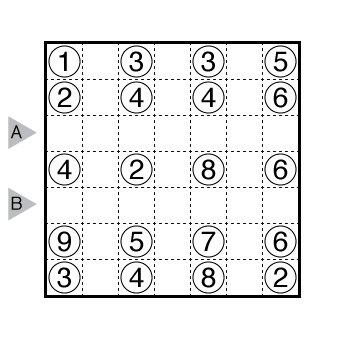PDF

Theme: Aisles

Author/Opus: This is the 177th puzzle from Thomas Snyder, aka Dr. Sudoku.

Rules: Divide the grid into some regions formed of edge-adjacent squares. Each cell is part of one region, and each region should contain exactly two given numbers. Each region must have an area that is strictly between those numbers (This means, for two number clues A and B with A < B, the area C fulfills A < C < B).Answer String: Enter the number of cells in each connected group (between bold lines) in the marked rows. For the example above, this is “34,121111,4111”.

Time Standards (highlight to view): Grandmaster = 0:45, Master = 1:15, Expert = 2:30

Note: Araf is a relatively new puzzle for us on GMPuzzles. Follow this link for other Araf.